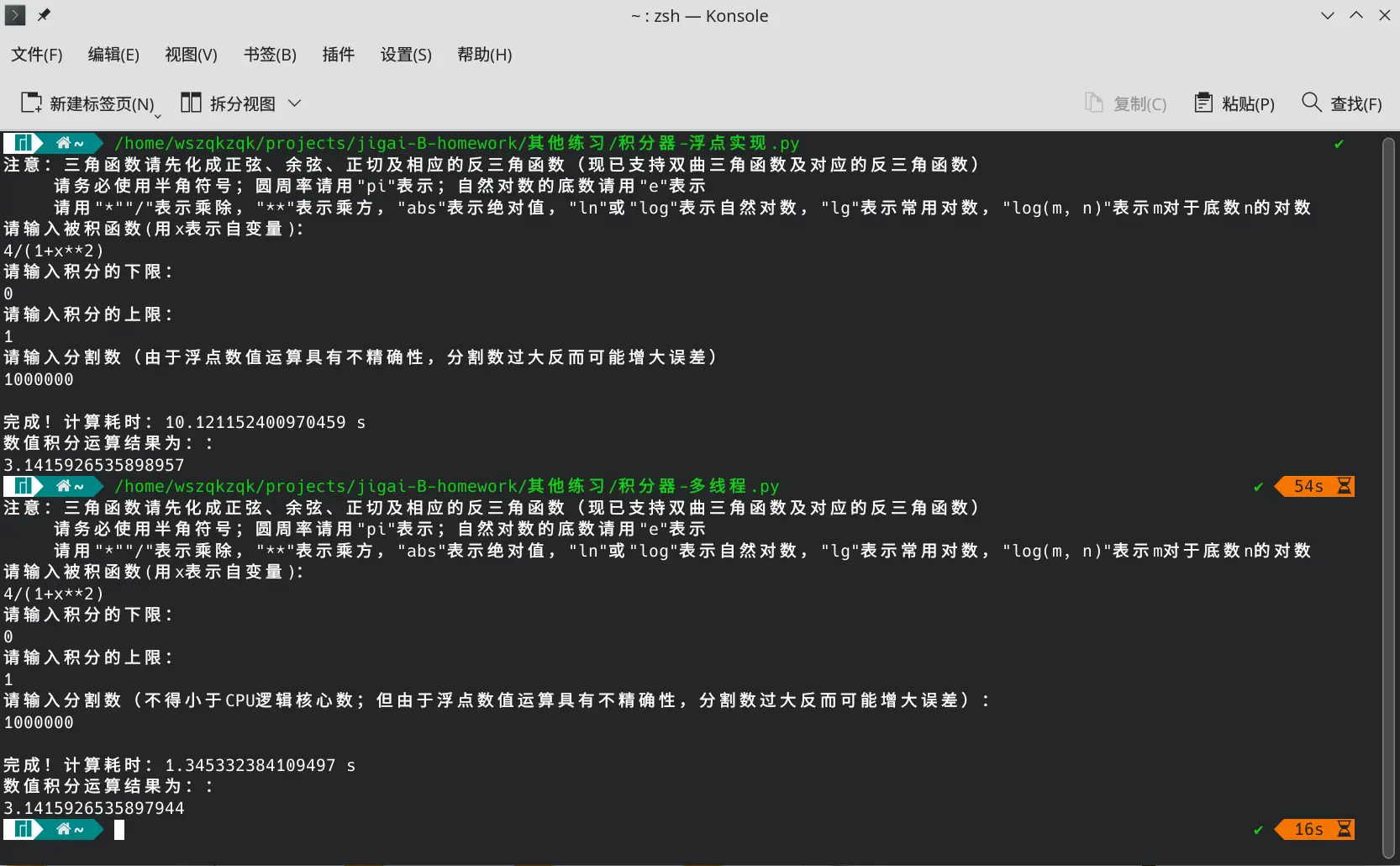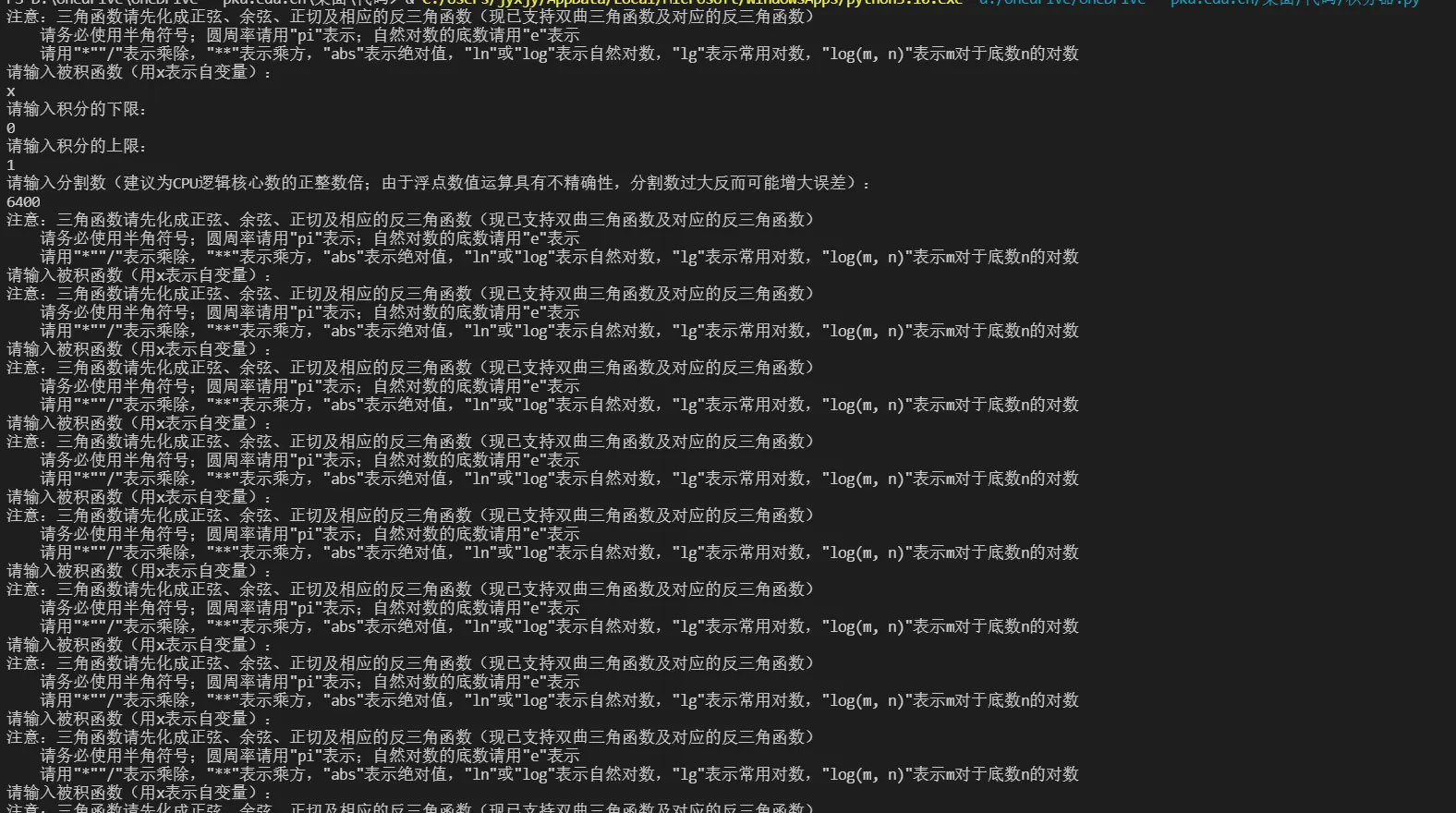# Python多进程与Windows下exe封装的踩坑实践

## multiprocessing、pyinstaller、nuitka的尝试

Posted by wszqkzqk on January 20, 2022

## 多进程踩坑经历

### 代码

#### 单进程的代码

#!/usr/bin/env python3

from timeit import default_timer as time
from math import *
# 按照习惯名称对部分函数定义别名

def ln(x):
return log(x)
def lg(x):
return log(x, 10)
def arcsin(x):
return asin(x)
def arccos(x):
return acos(x)
def arctan(x):
return atan(x)
def arcsinh(x):
return asinh(x)
def arccosh(x):
return acosh(x)
def arctanh(x):
return atanh(x)
print('注意：三角函数请先化成正弦、余弦、正切及相应的反三角函数（现已支持双曲三角函数及对应的反三角函数）\n     请务必使用半角符号；圆周率请用"pi"表示；自然对数的底数请用"e"表示\n     请用"*""/"表示乘除，"**"表示乘方，"abs"表示绝对值，"ln"或"log"表示自然对数，"lg"表示常用对数，"log(m, n)"表示m对于底数n的对数\n请输入被积函数（用x表示自变量）：')   # 预备信息

fx = input()
print('请输入积分的下限：')
start = eval(input())   # 使用eval函数，支持输入表达式

print('请输入积分的上限：')
end = eval(input())
print('请输入分割数（由于浮点数值运算具有不精确性，分割数过大反而可能增大误差）')
block = int(input())
calcstart = time()  # 输入结束，计算开始，计时

length = (end - start) / block
halflength = length / 2
out = 0
x = start
temp2 = eval(fx)    # 初始化x与temp2，以便后续让temp0调用上一次的temp2的值，可以减小运算量

for i in range(1, block + 1):   # 积分运算，辛普森法

temp0 = temp2
x += halflength
temp1 = eval(fx)
x = start + i*length

temp2 = eval(fx)
temp = (temp0 + 4*temp1 + temp2) / 6
out += temp*length
print('\n完成！计算耗时：{}s'.format(time() - calcstart))
print('数值积分运算结果为：')
print(out)


### 改为多进程

• 方便批量建立进程
• 内置了Pool(n).map()（仅支持一个参数）和Pool(n).starmap()（支持多个参数）函数，是map()函数的进程池版本，可以多进程地对可迭代对象进行目标函数操作，并返回map对象
• 返回对象处理方便，只需要套一层sum()函数就能得到最终结果

#### 定义积分函数

# 用于积分的函数

def integration(blockstart, blockend):
out = 0
x = start + blockstart*length
temp2 = eval(fx)    # 初始化x与temp2，以便后续让temp0调用上一次的temp2的值，可以减小运算量

for i in range(blockstart + 1, blockend + 1):
temp0 = temp2
x += halflength
temp1 = eval(fx)
x = start + i*length

temp2 = eval(fx)
temp = (temp0 + 4*temp1 + temp2) / 6
out += temp*length
return out


#### 进程数与区间分段

from os import cpu_count
n = cpu_count() # 默认为设备的逻辑核心数

tile = int(block / n)

# 进行分段，以便分进程计算

tilestart = 0
obj = 
for i in range(n):
tilestart += tile
obj.append(tilestart)


#### 初版整体代码

from timeit import default_timer as time
from os import cpu_count
n = cpu_count()    # 默认为设备的逻辑核心数

from multiprocessing import Pool
from math import *
def ln(x):
return log(x)
def lg(x):
return log(x, 10)
def arcsin(x):
return asin(x)
def arccos(x):
return acos(x)
def arctan(x):
return atan(x)
def arcsinh(x):
return asinh(x)
def arccosh(x):
return acosh(x)
def arctanh(x):
return atanh(x)
print('注意：三角函数请先化成正弦、余弦、正切及相应的反三角函数（现已支持双曲三角函数及对应的反三角函数）\n     请务必使用半角符号；圆周率请用"pi"表示；自然对数的底数请用"e"表示\n     请用"*""/"表示乘除，"**"表示乘方，"abs"表示绝对值，"ln"或"log"表示自然对数，"lg"表示常用对数，"log(m, n)"表示m对于底数n的对数\n请输入被积函数（用x表示自变量）：')
fx = input()
print('请输入积分的下限：')
start = eval(input())
print('请输入积分的上限：')
end = eval(input())
print('请输入分割数（建议为CPU逻辑核心数的正整数倍；由于浮点数值运算具有不精确性，分割数过大反而可能增大误差）：')
block = int(input())
calcstart = time()
length = (end - start) / block
halflength = length / 2
tile = int(block / n)

# 用于积分的函数

def integration(blockstart):
out = 0
x = start + blockstart*length
temp2 = eval(fx)    # 初始化x与temp2，以便后续让temp0调用上一次的temp2的值，可以减小运算量

if blockstart == tilestart:
blockend = block
else:
blockend = blockstart + tile
for i in range(blockstart + 1, blockend + 1):
temp0 = temp2
x += halflength
temp1 = eval(fx)
x = start + i*length
temp2 = eval(fx)
temp = (temp0 + 4*temp1 + temp2) / 6
out += temp*length
return out

# 进行分段，以便分线程计算

tilestart = 0
obj = 
for i in range(n):
tilestart += tile
obj.append(tilestart)

# 分线程计算

with Pool(n) as pool:
out = sum(pool.map(integration, obj))

# 显示输出

print('\n完成！计算耗时：{} s'.format(time() - calcstart))
print('数值积分运算结果为：')
print(out)


### 踩坑：初版代码运行结果Linux下单进程与多进程程序效率对比：在100,0000次分割的高计算量下，多进程程序效率是单进程程序的7.5倍 1Windows平台下各个子进程从头执行程序，并非只执行目标函数

### 问题修复后的情况

#### 代码

#!/usr/bin/env python3

from math import *
def ln(x):
return log(x)
def lg(x):
return log(x, 10)
def arcsin(x):
return asin(x)
def arccos(x):
return acos(x)
def arctan(x):
return atan(x)
def arcsinh(x):
return asinh(x)
def arccosh(x):
return acosh(x)
def arctanh(x):
return atanh(x)
if __name__ == '__main__':
from platform import system
if system() == 'Windows':
print('检测到您在Windows平台下，Windows下多进程初始化耗时较久，当计算量过小时无法发挥性能优势')
from timeit import default_timer as time
from os import cpu_count
n = cpu_count() # 默认为设备的逻辑核心数

from multiprocessing import Pool
print('       多进程积分器 <一个简单的多进程数值积分工具>\n    Copyright (C) 2021-2022 星外之神 <wszqkzqk@qq.com>\n\n注意：三角函数请先化成正弦、余弦、正切及相应的反三角函数（现已支持双曲三角函数及对应的反三角函数）\n    请务必使用半角符号；圆周率请用"pi"表示；自然对数的底数请用"e"表示\n    请用"*""/"表示乘除，"**"表示乘方，"abs"表示绝对值，"ln"或"log"表示自然对数，"lg"表示常用对数，"log(m, n)"表示m对于底数n的对数\n请输入被积函数（用x表示自变量）：')
fx = input()
print('请输入积分的下限：')
start = eval(input())
print('请输入积分的上限：')
end = eval(input())
print('请输入分割数（建议为CPU逻辑核心数的正整数倍；由于浮点数值运算具有不精确性，分割数过大反而可能增大误差）：')
block = int(input())
calcstart = time()
length = (end - start) / block
halflength = length / 2
tile = int(block / n)

# 用于积分的函数

def integration(blockstart, blockend, start, length, halflength, fx):
out = 0
x = start + blockstart*length
temp2 = eval(fx)    # 初始化x与temp2，以便后续让temp0调用上一次的temp2的值，可以减小运算量

for i in range(blockstart + 1, blockend + 1):
temp0 = temp2
x += halflength
temp1 = eval(fx)
x = start + i*length

temp2 = eval(fx)
temp = (temp0 + 4*temp1 + temp2) / 6
out += temp*length
return out

if __name__ == '__main__':

# 进行分段，以便分进程计算

tilestart = 0
obj = []
for i in range(n - 1):
tileend = tilestart + tile
obj.append((tilestart, tileend, start, length, halflength, fx))
tilestart = tileend
obj.append((tilestart, block, start, length, halflength, fx))

# 分进程计算

with Pool(n) as pool:
out = sum(pool.starmap(integration, obj))

# 显示输出

print('\n完成！计算耗时：{}s'.format(time() - calcstart))
print('数值积分运算结果为：')
print(out)


#### 性能状况

• Windows下进程资源消耗较Linux(POSIX)大，建立进程耗时长（经过验证，空载的时间消耗主要来自建立进程池这一步）
• 因为Windows下的spawn是直接新建一个进程，而POSIX的fork是利用写时复制技术在原有进程基础上建立新的进程，spawn的资源占用比fork大得多
• Windows下官方版Python是用MSVC编译的，性能较GCC编译的差（虚拟机（8线程）Windows系统中用MinGW编译的Python运行该程序的速度甚至比实体机（16线程）上的快）

## Windows下exe的编译

### pyinstaller

pyinstaller编译的并非是机器码文件，它只是将代码编译为python字节码（.pyc文件），然后将其与解释器和所需要的库封装到一起，因此也不依赖C编译器。然而，我并没有搞清这个pyinstaller封装的是所在Python环境的解释器还是自身库所携带的Python解释器（懒～），所以其实并不一定有性能提升### 解决办法

• 此外，假如该程序没有输入这一步阻隔后续步骤，由于每次建立进程都会完整启动一遍程序，将会无限建立进程，导致内存泄漏，把程序变为“内存炸弹”
• 因此用pyinstaller编译python多进程程序风险很大，一不小心就做成了一个病毒般的东西

• 原理上pyinstaller是将python.dll目标.pyc文件封装在一起，因此实际上pyinstaller并不能显著优化python程序的速度

### nuitka

• 然而，这里的二进制文件并非直接由机器执行，而仍然是调用python解释器进行解释，因此并不会拥有C语言的运行效率
• Nuitka的编译主要是把python的PyObject抽离到C中
• 由于Nuitka在编译过程中有所优化，因此Nuitka编译的程序相比编译前有一定的性能提升
• 根据我的简单测试，四则运算中整数、浮点运算均有越2~3倍的性能提升，其中浮点运算性能提升更加明显

#### 踩坑：编译器支持

• 2022.05.31更新：与nuitka作者取得联系后，作者告知可以用--mingw64 --clang来使用MSYS2（MingW-w64）中的Clang

#### 踩坑：Python安装来源

• 2022.02.17更新：现在从Msys2官方源pacman安装的Nuitka已经可用了，但是不能在bashzshfish这样的Msys2终端中执行，否则会因为错误调用/usr/lib中的库而编译失败（应当调用的是/mingw64/bin中的库，不知道为什么在Msys2终端中会出现这个错误，可能是Msys2终端指定了默认值？？？）
• 2022.05.31更新：可以取消bashzshfish启动的--login参数，或者直接在cmdpowershellpwsh中启动bashzshfish来避免这一问题

#### 编译

nuitka除了依赖C编译器外，还需要其他几个库的支持，好在nuitka在运行过程中可以自动解决依赖问题，因此也并不算麻烦；我编译多进程积分器的命令如下：

nuitka --mingw64 --standalone --onefile --show-progress --show-memory --enable-plugin=multiprocessing --windows-icon-from-ico=target.ico --output-dir=out targetfile.py


MSYS2的各个shell可能无法识别Windows的.bat文件，需要将命令改为：

python -m nuitka --mingw64 --standalone --onefile --show-progress --show-memory --enable-plugin=multiprocessing --windows-icon-from-ico=target.ico --output-dir=out targetfile.py


#### 代码

#!/usr/bin/env python3

from math import *
def ln(x):
return log(x)
def lg(x):
return log(x, 10)
def arcsin(x):
return asin(x)
def arccos(x):
return acos(x)
def arctan(x):
return atan(x)
def arcsinh(x):
return asinh(x)
def arccosh(x):
return acosh(x)
def arctanh(x):
return atanh(x)
if __name__ == '__main__':
from timeit import default_timer as time
from os import cpu_count
n = cpu_count() # 默认为设备的逻辑核心数

from multiprocessing import Pool
print('       多进程积分器 <一个简单的多进程数值积分工具>\n    Copyright (C) 2021-2022 星外之神 <wszqkzqk@qq.com>\n\n注意：三角函数请先化成正弦、余弦、正切及相应的反三角函数（现已支持双曲三角函数及对应的反三角函数）\n    请务必使用半角符号；圆周率请用"pi"表示；自然对数的底数请用"e"表示\n    请用"*""/"表示乘除，"**"表示乘方，"abs"表示绝对值，"ln"或"log"表示自然对数，"lg"表示常用对数，"log(m, n)"表示m对于底数n的对数\n请输入被积函数（用x表示自变量）：')
fx = input()
print('请输入积分的下限：')
start = eval(input())
print('请输入积分的上限：')
end = eval(input())
print('请输入分割数（建议为CPU逻辑核心数的正整数倍；由于浮点数值运算具有不精确性，分割数过大反而可能增大误差）：')
block = int(input())
calcstart = time()
length = (end - start) / block
halflength = length / 2
tile = int(block / n)

# 用于积分的函数

def integration(blockstart, blockend, start, length, halflength, fx):
out = 0
x = start + blockstart*length
temp2 = eval(fx)    # 初始化x与temp2，以便后续让temp0调用上一次的temp2的值，可以减小运算量

for i in range(blockstart + 1, blockend + 1):
temp0 = temp2
x += halflength
temp1 = eval(fx)
x = start + i*length

temp2 = eval(fx)
temp = (temp0 + 4*temp1 + temp2) / 6
out += temp*length
return out

if __name__ == '__main__':

# 进行分段，以便分进程计算

tilestart = 0
obj = []
for i in range(n - 1):
tileend = tilestart + tile
obj.append((tilestart, tileend, start, length, halflength, fx))
tilestart = tileend
obj.append((tilestart, block, start, length, halflength, fx))

# 分进程计算

with Pool(n) as pool:
out = sum(pool.starmap(integration, obj))

# 显示输出

print('\n完成！计算耗时：{}s'.format(time() - calcstart))
print('数值积分运算结果为：')
print(out)

input('\n请按回车键退出')


#### 性能

nuitka下调用MinGW编译的多进程积分器（点此下载）性能相比于MSVC编译的Python中运行的程序有一定的提高，接近在MinGW编译的Python中运行的水平，但是仍然与Linux下的性能表现有较大差距

• 2022.01.21更新：这个积分器在Windows平台的运行速度受编译器影响似乎不是很大，让程序程序运行缓慢的真正原因可能是使用来自Microsoft Store中的Python；Python官网上的Python也是由MSVC编译而成，但是性能与MinGW编译的Python并没有明显差距（从Python官网上下载的Python空载启动时间约0.35s，100,0000次分割运算时间约2s）。
• 2022.02.15更新：奇怪的是，wine运行Nuitka所打包的程序耗时空载耗时比Windows还短（仅需要0.3s），100,0000次分割运算时间仍约2s，与Windows下的表现接近
• 2022.05.14更新：目前推测性能优化不明显的原因是该积分器最大的时间消耗在eval一步字符串到表达式的转化，而这步操作对于编译器而言很难优化，所以耗时其实都差不多

## 关于代码的优化（2022.5.16更新）

### 代码

#### 单进程

#!/usr/bin/env python

#   积分器 <一个简单的数值积分工具>
#   Copyright (C) 2021-2022 星外之神 <wszqkzqk@qq.com>
#
#   This program is free software: you can redistribute it and/or modify
#   the Free Software Foundation, either version 3 of the License, or
#   (at your option) any later version.
#
#   This program is distributed in the hope that it will be useful,
#   but WITHOUT ANY WARRANTY; without even the implied warranty of
#   MERCHANTABILITY or FITNESS FOR A PARTICULAR PURPOSE.  See the
#   GNU General Public License for more details.
#
#   You should have received a copy of the GNU General Public License
#   along with this program.  If not, see <https://www.gnu.org/licenses/>.

from timeit import default_timer as time
from math import *
def ln(x):
return log(x)
def lg(x):
return log(x, 10)
def arcsin(x):
return asin(x)
def arccos(x):
return acos(x)
def arctan(x):
return atan(x)
def arcsinh(x):
return asinh(x)
def arccosh(x):
return acosh(x)
def arctanh(x):
return atanh(x)
print('''       积分器 <一个简单的数值积分工具>

请务必使用半角符号；圆周率请用"pi"表示；自然对数的底数请用"e"表示
请用"*""/"表示乘除，"**"表示乘方，"abs"表示绝对值，"ln"或"log"表示自然对数，"lg"表示常用对数，"log(m, n)"表示m对于底数n的对数

fx = compile(input(), '', 'eval')
print('请输入积分的下限：')
start = eval(input())
print('请输入积分的上限：')
end = eval(input())
print('请输入分割数（由于浮点数值运算具有不精确性，分割数过大反而可能增大误差）')
block = int(input())
calcstart = time()
length = (end - start) / block
halflength = length / 2
out = 0
x = start
temp2 = eval(fx)    # 初始化x与temp2，以便后续让temp0调用上一次的temp2的值，可以减小运算量

for i in range(1, block + 1):
temp0 = temp2
x += halflength
temp1 = eval(fx)
x = start + i*length
temp2 = eval(fx)
temp = (temp0 + 4*temp1 + temp2) / 6
out += temp*length
print('\n完成！计算耗时：{}s'.format(time() - calcstart))
print('数值积分运算结果为：')
print(out)

input('\n请按回车键退出...')


#### 多进程

#!/usr/bin/env python3

from math import *
def ln(x):
return log(x)
def lg(x):
return log(x, 10)
def arcsin(x):
return asin(x)
def arccos(x):
return acos(x)
def arctan(x):
return atan(x)
def arcsinh(x):
return asinh(x)
def arccosh(x):
return acosh(x)
def arctanh(x):
return atanh(x)
if __name__ == '__main__':
from timeit import default_timer as time
from os import cpu_count
n = cpu_count() # 默认为设备的逻辑核心数

from multiprocessing import Pool
print('       多进程积分器 <一个简单的多进程数值积分工具>\n    Copyright (C) 2021-2022 星外之神 <wszqkzqk@qq.com>\n\n注意：三角函数请先化成正弦、余弦、正切及相应的反三角函数（现已支持双曲三角函数及对应的反三角函数）\n    请务必使用半角符号；圆周率请用"pi"表示；自然对数的底数请用"e"表示\n    请用"*""/"表示乘除，"**"表示乘方，"abs"表示绝对值，"ln"或"log"表示自然对数，"lg"表示常用对数，"log(m, n)"表示m对于底数n的对数\n请输入被积函数（用x表示自变量）：')
fx = input()
print('请输入积分的下限：')
start = eval(input())
print('请输入积分的上限：')
end = eval(input())
print('请输入分割数（建议为CPU逻辑核心数的正整数倍；由于浮点数值运算具有不精确性，分割数过大反而可能增大误差）：')
block = int(input())
calcstart = time()
length = (end - start) / block
halflength = length / 2
tile = int(block / n)

# 用于积分的函数

def integration(blockstart, blockend, start, length, halflength, func):
out = 0
x = start + blockstart*length
fx = compile(func, '', 'eval')
temp2 = eval(fx)    # 初始化x与temp2，以便后续让temp0调用上一次的temp2的值，可以减小运算量

for i in range(blockstart + 1, blockend + 1):
temp0 = temp2
x += halflength
temp1 = eval(fx)
x = start + i*length
temp2 = eval(fx)
temp = (temp0 + 4*temp1 + temp2) / 6
out += temp*length
return out

if __name__ == '__main__':

# 进行分段，以便分进程计算

tilestart = 0
obj = []
for i in range(n - 1):
tileend = tilestart + tile
obj.append((tilestart, tileend, start, length, halflength, fx))
tilestart = tileend
obj.append((tilestart, block, start, length, halflength, fx))

# 分进程计算

with Pool(n) as pool:
out = sum(pool.starmap(integration, obj))

# 显示输出

print('\n完成！计算耗时：{}s'.format(time() - calcstart))
print('数值积分运算结果为：')
print(out)

input('\n请按回车键退出')


#### 自动判断

Windows编译文件下载地址：点击这里下载（封装python 3.10环境，MSVC编译）

from math import *
from timeit import default_timer as time
from os import cpu_count
from os import name as systemName
from multiprocessing import Pool

n = cpu_count() # 默认为设备的逻辑核心数

def ln(x):
return log(x)
def lg(x):
return log(x, 10)
def arcsin(x):
return asin(x)
def arccos(x):
return acos(x)
def arctan(x):
return atan(x)
def arcsinh(x):
return asinh(x)
def arccosh(x):
return acosh(x)
def arctanh(x):
return atanh(x)
def integration(blockstart, blockend, start, length, halflength, func):
out = 0
x = start + blockstart*length
fx = compile(func, '', 'eval')
temp2 = eval(fx)
for i in range(blockstart + 1, blockend + 1):
temp0 = temp2
x += halflength
temp1 = eval(fx)
x = start + i*length
temp2 = eval(fx)
temp = (temp0 + 4*temp1 + temp2) / 6
out += temp*length
return out

if __name__ == '__main__':
print('''       积分器 <一个简单的数值积分工具（支持自动切换多进程）>

请务必使用半角符号；圆周率请用"pi"表示；自然对数的底数请用"e"表示
请用"*""/"表示乘除，"**"表示乘方，"abs"表示绝对值，"ln"或"log"表示自然对数，"lg"表示常用对数，"log(m, n)"表示m对于底数n的对数
Windows下多进程初始化耗时较久，默认在分割数不小于100,0000时才启用多进程计算，其他平台则在任意分割数下均默认启用多进程计算

fx = input()
while True:
try:
x = start = eval(input('请输入积分的下限：\n'))
break
except Exception:
print("错误！请检查后重新输入！")
while True:
try:
end = eval(input('请输入积分的上限：\n'))
break
except Exception:
print("错误！请检查后重新输入！")
while True:
try:
block = int(input('请输入分割数（建议为CPU逻辑核心数的正整数倍；由于浮点数值运算具有不精确性，分割数过大反而可能增大误差）：\n'))
break
except Exception:
print("错误！请检查后重新输入！")
while True:
try:
func = compile(fx, '', 'eval')
temp2 = eval(func)    # 初始化x与temp2，以便后续让temp0调用上一次的temp2的值，可以减小运算量

break
except Exception:
print("被积函数输入错误！请检查后重新输入！")
fx = input("请输入被积函数（用x表示自变量）：\n")
calcstart = time()
length = (end - start) / block
halflength = length / 2
tile = int(block / n)

# 分割数较大时多进程计算

if (block >= 1000000) or (systemName != 'nt'):
# 进行分段，以便分进程计算

tilestart = 0
obj = []
for i in range(n - 1):
tileend = tilestart + tile
obj.append((tilestart, tileend, start, length, halflength, fx))
tilestart = tileend
obj.append((tilestart, block, start, length, halflength, fx))

with Pool(n) as pool:
out = sum(pool.starmap(integration, obj))

print('\n完成！计算耗时：{}s'.format(time() - calcstart))
print('数值积分运算结果为：')
print(out)

input('\n请按回车键退出...')

else:
out = 0

for i in range(1, block + 1):
temp0 = temp2
x += halflength
temp1 = eval(func)
x = start + i*length
temp2 = eval(func)
temp = (temp0 + 4*temp1 + temp2) / 6
out += temp*length
print('\n完成！计算耗时：{}s'.format(time() - calcstart))
print('数值积分运算结果为：')
print(out)

input('\n请按回车键退出...')

1. 所有数据均由搭载AMD 锐龙5800H的联想小新Pro 16在35w TDP功耗的均衡模式下测试出  2 3